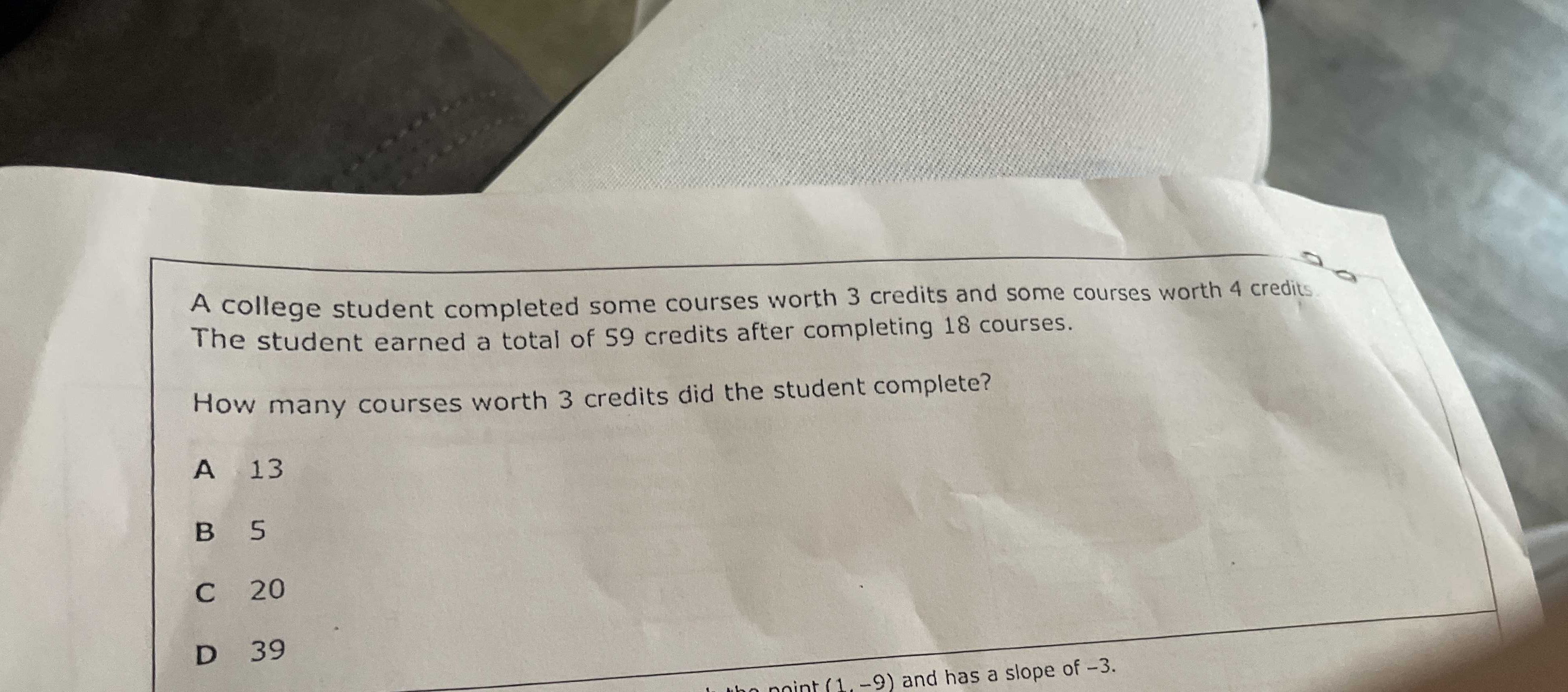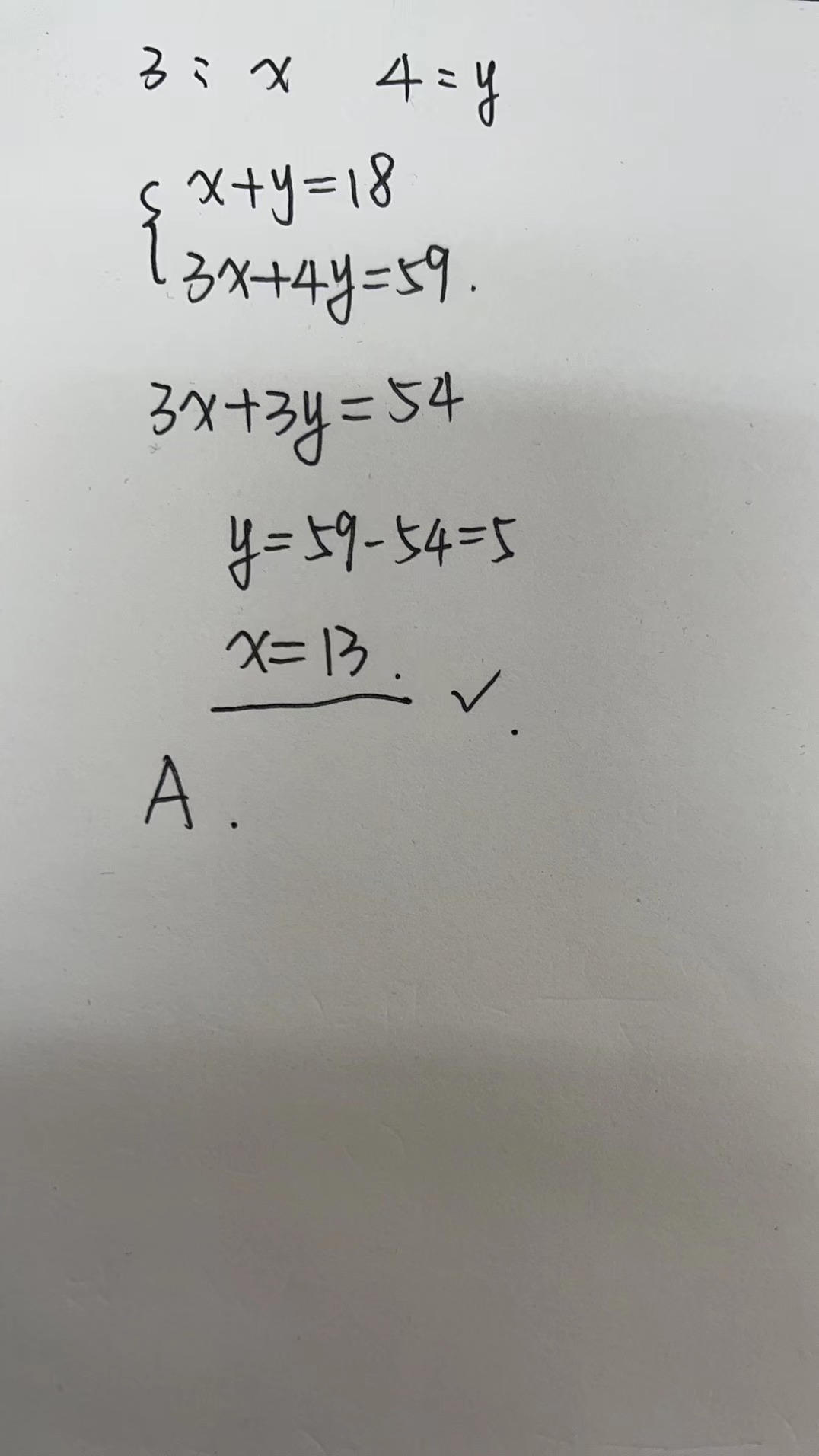### ¿Todavía tienes preguntas de matemáticas?

Pregunte a nuestros tutores expertos
Algebra
PreguntaA college student completed some courses worth $$3$$ credits and some courses worth $$4$$ credits The student earned a total of $$59$$ credits after completing $$18$$ courses. How many courses worth $$3$$ credits did the student complete? A $$13$$ B $$5$$ C $$20$$ D $$39$$